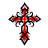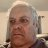# Sell Relative Strength Index (sRSI) Indicator for ThinkorSwim#### netarchitech

##### Well-known member
VIP
I'm trying to convert a Tradestation ELS into Thinkscript. Here is the Tradestation code:

Rich (BB code):
``````inputs:
Length(20);

vars:
srsi(0),
srsin(0),
k(0);

srsin = 0;
For k = 0 to Length - 1
Begin
Value1 = absvalue(close[k] - open[k];
Value2 = absvalue(high[k] - low[k];
If value2 = 0 then value3 = 0
Else
Value3 = value1/value2;
srsin = srsin + value3;
End;

srsi = srsin/length;

plot1(0.38);
plot2(srsi);
plot3(-0.38);
plot4(0);
If srsi > 0 then Setplotcolor(2,green);
If srsi < 0 then Setplotcolor(2,red);
If srsi >= -0.05 and srsi <= 0.05 then Setplotcolor(2,yellow);

Setplotcolor(1,darkred);
Setplotcolor(3,darkgreen);
Setplotcolor(4,yellow);``````

Here is my attempt at converting it to Thinkscript:

Rich (BB code):
``````declare lower;

input Length = 20;

def srsi = 0;
def srsin = 0;
def k = 0;

plot iteration = fold k = 0 to Length - 1;

def Value1 = absvalue(close[k] - open[k]);
def Value2 = absvalue(high[k] - low[k]);
def Value = if value2 == 0 then value3 == 0 else value3 == value1 / value2;

def srsin = srsin + value3;

def srsi = srsin / Length;

plot line1 = 0.38;
line1.SetDefaultColor(Color.DARK_RED);
line1.HideTitle();
line1.HideBubble();

plot line2 = srsi;
line2.AssignValueColor(if srsi > 0 then Color.GREEN
else if srsi < 0 then Color.RED
else if srsi >= -0.05 and srsi <= 0.05 then Color.YELLOW
else Color.GRAY);
line2.SetLineWeight(2);
line2.HideTitle();

plot line3 = -0.38;
line3.SetDefaultColor(Color.DARK_GREEN);
line3.HideTitle();
line3.HideBubble();

plot ZeroLine = 0;
ZeroLine.SetDefaultColor(Color.ORANGE);
ZeroLine.HideTitle();
ZeroLine.HideBubble();``````

The Thinkscript compiler says the fold statement is invalid. This is the first time I've attempted to work with a fold statement.

Any assistance would be greatly appreciated. Thanks in advance...

Last edited:#### netarchitech

##### Well-known member
VIPSuccess! For those of you whom might be interested in taking the converted code for a test drive:

Rich (BB code):
``````declare lower;

input len = 7; #changed from the setting of 20 per the instructions of the original author

def srsin;
def value1 = close - open;
def value2 = high - low;
def value3 = if value2 == 0 then 0
else value1 / value2;

srsin = Sum(value3, len);

plot srsi = srsin / len;
srsi.assignValueColor(if srsi > 0.05 then Color.GREEN
else if srsi<-0.05 then color.red
else color.yellow);

plot l1 = 0.38 ;
l1.SetDefaultColor(Color.DARK_RED);

plot l2 = -0.38;
l2.SetDefaultColor(Color.DARK_GREEN);

plot zline = 0;
zline.SetDefaultColor(Color.ORANGE);``````

Last edited:
•skynetgen and korygill#### BenTen

Staff
VIP
@netarchitech Glad you got it work &#128515;

Last edited:#### netarchitech

##### Well-known member
VIP
@BenTen Yeah, me too... It was a good learning experienceLast edited:#### BenTen

Staff
VIP
@netarchitech What is this indicator called? I'll help you change the title and move it into the proper category?

Last edited:#### netarchitech

##### Well-known member
VIP
@BenTen ThanksIt's called sRSI...

Last edited:
J

#### john3

##### Active member
2019 Donor
What are the upper indicators? Would you please post links for them?

Thank you.

Last edited:#### netarchitech

##### Well-known member
VIP
@john3
Good Luck and Good TradingLast edited:
W

##### New member
VIP#### BenTen

Staff
VIP
Are you referring to the sRSI being shared in this post? It's right above.

W

##### New member
VIP
Are you referring to the sRSI being shared in this post? It's right above.

Thank you. I downloaded it and its working. I didn't notice it.#### netarchitech

##### Well-known member
VIP
•D

.

Last edited:
M

#### martyrjohn

##### Member
@netarchitech can you explain how to use this#### Rojo Grande

##### Member
VIP
Hello,

I am trying to piece together a study that shows the avg. srsi of two tickers. I have posted below what I have pieced together, but just doesn't appear correct. It could use a good editing above my cut and paste skills. Any help is appreciated, thank you.

Code:
``````declare lower;

input len = 7; #changed from the setting of 20 per the instructions of the original author

input ticker_1 = "COMP";
input ticker_2 = "\$DJI";

def srsin_1;
def value1 = close(ticker_1) - open;
def value2 = high - low;
def value3 = if value2 == 0 then 0
else value1 / value2;

def srsin_2;
def value4 = close(ticker_2) - open;
def value5 = high - low;
def value6 = if value5 == 0 then 0
else value4 / value5;

srsin_1 = Sum(value3, len);
srsin_2 = sum(value6, len);

plot srsi = (srsin_1 + srsin_2) / 2;
srsi.assignValueColor(if srsi > 0.05 then Color.GREEN
else if srsi<-0.05 then color.red
else color.yellow);

plot l1 = 0.38 ;
l1.SetDefaultColor(Color.DARK_RED);

plot l2 = -0.38;
l2.SetDefaultColor(Color.DARK_GREEN);

plot zline = 0;
zline.SetDefaultColor(Color.ORANGE);``````HOT ZONE - RSI with IV Percentile: Buy Stock or Sell Put Options Signal Indicators 153Strategy Sell before Buy Questions 7